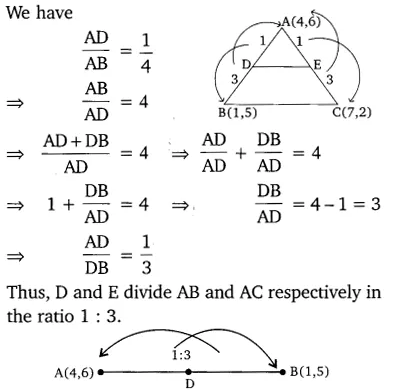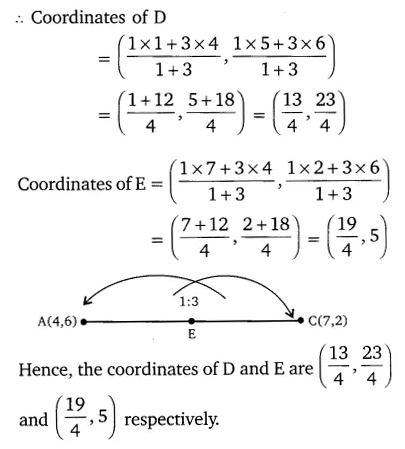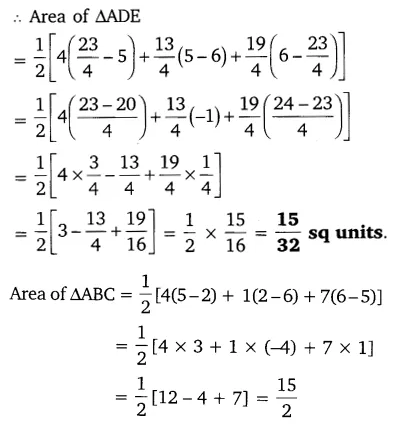# The vertices of a VABC are A(4, 6), B(1, 5) and C(7, 2)

The vertices of a VABC are A(4, 6), B(1, 5) and C(7, 2). A line is drawn to intersect sides AB and AC at D and E respectively. such that AD/AB = AE/AC = 1/4… calculate the area of the ∆ADe and compare it with the area of ∆ABC.